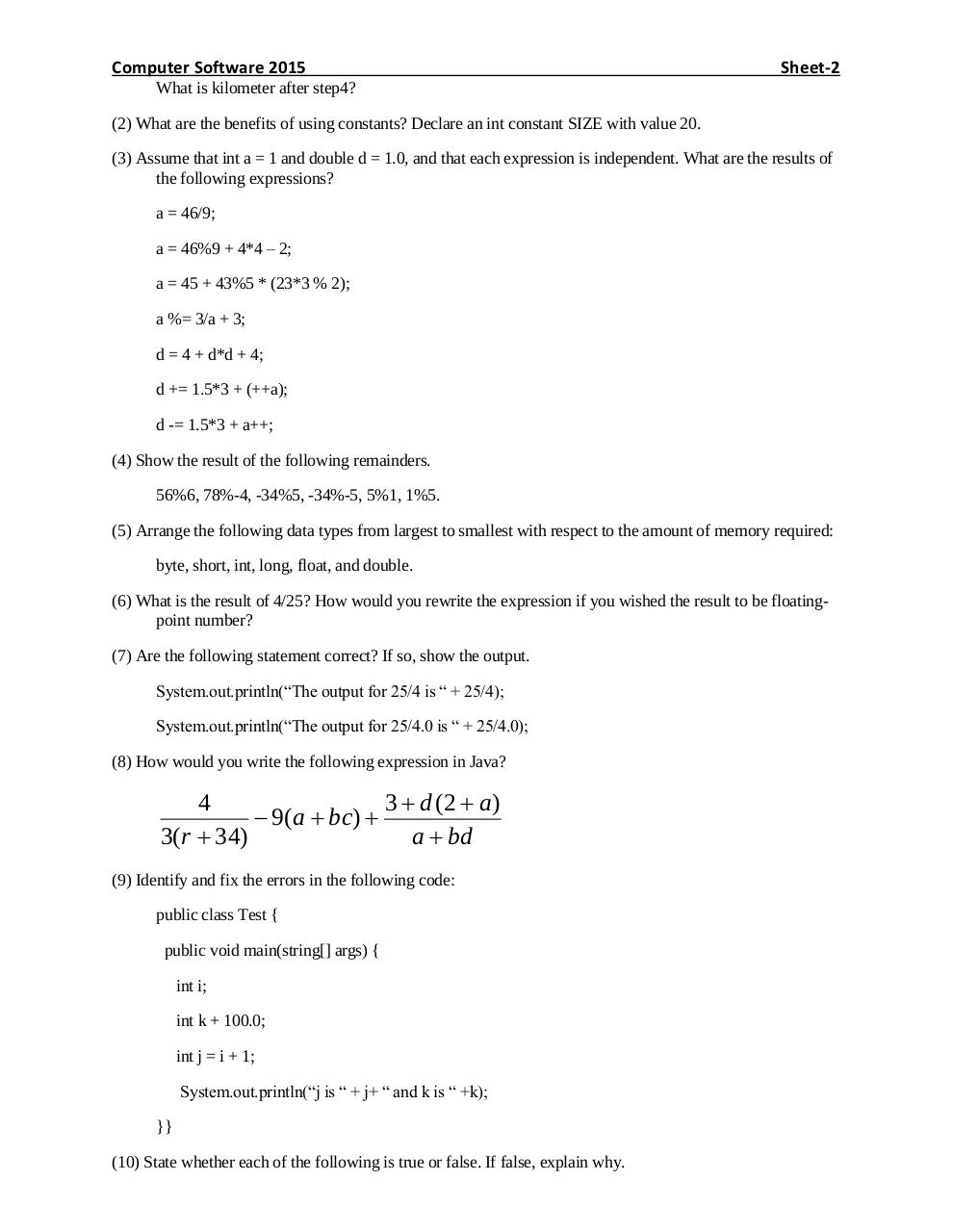# Sheet 2 2015.pdfPage 1 2 3 4 5 6 7

#### Text preview

Computer Software 2015

Sheet-2

What is kilometer after step4?
(2) What are the benefits of using constants? Declare an int constant SIZE with value 20.
(3) Assume that int a = 1 and double d = 1.0, and that each expression is independent. What are the results of
the following expressions?
a = 46/9;
a = 46%9 + 4*4 – 2;
a = 45 + 43%5 * (23*3 % 2);
a %= 3/a + 3;
d = 4 + d*d + 4;
d += 1.5*3 + (++a);
d -= 1.5*3 + a++;
(4) Show the result of the following remainders.
56%6, 78%-4, -34%5, -34%-5, 5%1, 1%5.
(5) Arrange the following data types from largest to smallest with respect to the amount of memory required:
byte, short, int, long, float, and double.
(6) What is the result of 4/25? How would you rewrite the expression if you wished the result to be floatingpoint number?
(7) Are the following statement correct? If so, show the output.
System.out.println(“The output for 25/4 is “ + 25/4);
System.out.println(“The output for 25/4.0 is “ + 25/4.0);
(8) How would you write the following expression in Java?

4
3  d (2  a)
 9(a  bc) 
3(r  34)
a  bd
(9) Identify and fix the errors in the following code:
public class Test {
public void main(string[] args) {
int i;
int k + 100.0;
int j = i + 1;
System.out.println(“j is “ + j+ “ and k is “ +k);
}}
(10) State whether each of the following is true or false. If false, explain why.